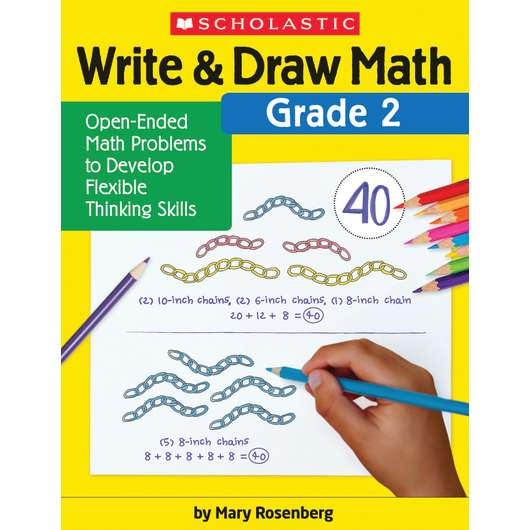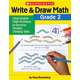•# Write & Draw Math, Grade 2

Product Number: SC-831438
\$11.99
There are many ways to answer the math problems in this book-and that’s exactly what children need to build their mathematica...
Quantity

There are many ways to answer the math problems in this book-and that’s exactly what children need to build their mathematical reasoning and critical thinking skills. They try different methods to solve the same math problem, using manipulatives, drawing, or writing. Because there is no single correct answer, each reproducible activity page can be reused multiple times as children’s math skills develop throughout the year. A fun and engaging way to promote mathematical thinking!
SC-831438